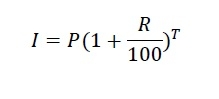# C Program for compound interest?

Here we will see how to get the compound interest by writing one C program. The logic is very easy. Here we need some parameters −

• P − Principle amount
• R − Rate of interest
• T − Time span

The compound interest formula is like below## Example

#include<stdio.h>
#include<math.h>
float compoundInterest(float P, float T, float R) {
return P*(pow(1+(R/100), T));
}
int main() {
float p, t, r;
printf("Enter Princple amount, rate of interest, and time: ");
scanf("%f%f%f", &p, &r, &t);
printf("Interest value: %f", compoundInterest(p, t, r));
}

## Output

Enter Princple amount, rate of interest, and time: 5000 7.5 3
Interest value: 6211.485352